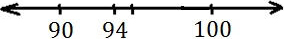# Rounding to Tens or Hundreds

#### Complete Python Prime Pack

9 Courses     2 eBooks

#### Artificial Intelligence & Machine Learning Prime Pack

6 Courses     1 eBooks

#### Java Prime Pack

9 Courses     2 eBooks

Rounding of a number means replacing it with another number that is nearly equal to it but easy to represent or write. For example 97 is rounded to 100.

If the rounding number is 4 or less it is round down. If the rounding number is 5 or more it is rounded up.

For example 31, 32, 33 and 34 round down to 30

35, 36, 37, 38 and 39 round up to 40.

Rules for Rounding to Tens or Hundreds

• To round a number to the nearest ten, the digit in ones place is considered. If > = 5, rounded up and if < 5 rounded down.

• To round a number to the nearest hundred, the digit in tens place is considered. If > = 5 rounded up and if < 5 rounded down.

Round 661 to the nearest hundred.

### Solution

Step 1:

We know that 661 lies between 600 and 700. To round 661 to the nearest hundred, we have to find if it closer to 600 or to 700.

Step 2:

Using the number line, we see that 661 is closer to 700.So we round up 661 to 700.

Step 3:

We look at the digit in tens place, 6. We find 6 is more 5. So we round up 661 to 700.

Round 94 to the nearest ten.

### Solution

Step 1:

To round 94 to nearest ten, we can use the number line to find if it is closer to 90 or to 100.From the number line we find that 94 is closer to 90. So, we round 94 to 90.

Step 2:

We can also look at the digit in ones place of 94; that is 4. Since 4 < 5, we round down 94 to 90